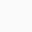# Percentage Class 7 Mathematics with sum explanation

This video contains following explanation.
2.Relation between percentage and fraction.
3.Conversion from percentage to fraction, percentage to decimal and vice versa.
4. Smaller unit of various quantity as a percentage of its larger unit. e.g. Paise as a percentage of Rupee, centimeter as a percentage of meter.
4. A quantity as a percentage of another quantity.

This video has explanation about these sums of percentage class 7 Mathematics.
1 Convert 7/16 into Percentage.
2. Convert 0.35 into percentage.
3. Covert 1:4 into percentage.
4. Convert 27 1/2 % into fraction.
5. Express 23 1/5 as decimal.
6. 16 2/3 % of length is 200 m find the length.
7. Find x . if x% of Rs 100 = 16.
8 . IF a number 500 is reduced to 425. Find the percentage reduction.
9. A sum of Rs 1000 first increases by 12 1/2%. Then resulting sum decreased by 10%. Find the resulting sum.

##### One thought on “Percentage Class 7 Mathematics with sum explanation”
1.Unknown says:

This article is very nice as well as very informative. I loved it. Fab!! I really appreciate sharing this. The video is really good and the way you have explained is really awesome. If you want essay related topic you can take help from https://essayreviewratings.com/